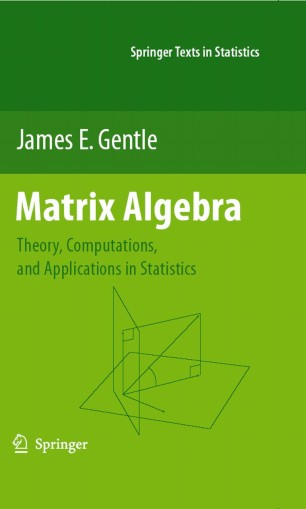# Matrix Algebra

## Theory, Computations, and Applications in Statistics

• James E. GentleTextbook

Part of the Springer Texts in Statistics book series (STS)

1. Front Matter
Pages i-xxii
2. ### Linear Algebra

1. Front Matter
Pages 1-1
2. Pages 9-39
3. Pages 41-143
4. Pages 145-171
5. Pages 173-200
6. Pages 201-239
7. Pages 241-257
3. ### Applications in Data Analysis

1. Front Matter
Pages 259-259
2. Pages 321-371
4. ### Numerical Methods and Software

1. Front Matter
Pages 373-373
2. Pages 375-427
3. Pages 429-443
4. Pages 445-477
5. Back Matter
Pages 479-528

### Introduction

Matrix algebra is one of the most important areas of mathematics for data analysis and for statistical theory. The first part of this book presents the relevant aspects of the theory of matrix algebra for applications in statistics. This part begins with the fundamental concepts of vectors and vector spaces, next covers the basic algebraic properties of matrices, then describes the analytic properties of vectors and matrices in the multivariate calculus, and finally discusses operations on matrices in solutions of linear systems and in eigenanalysis. This part is essentially self-contained.

The second part of the book begins with a consideration of various types of matrices encountered in statistics, such as projection matrices and positive definite matrices, and describes the special properties of those matrices. The second part also describes some of the many applications of matrix theory in statistics, including linear models, multivariate analysis, and stochastic processes. The brief coverage in this part illustrates the matrix theory developed in the first part of the book. The first two parts of the book can be used as the text for a course in matrix algebra for statistics students, or as a supplementary text for various courses in linear models or multivariate statistics.

The third part of this book covers numerical linear algebra. It begins with a discussion of the basics of numerical computations, and then describes accurate and efficient algorithms for factoring matrices, solving linear systems of equations, and extracting eigenvalues and eigenvectors. Although the book is not tied to any particular software system, it describes and gives examples of the use of modern computer software for numerical linear algebra. This part is essentially self-contained, although it assumes some ability to program in Fortran or C and/or the ability to use R/S-Plus or Matlab. This part of the book can be used as the text for a course in statistical computing, or as a supplementary text for various courses that emphasize computations.

The book includes a large number of exercises with some solutions provided in an appendix.

James E. Gentle is University Professor of Computational Statistics at George Mason University. He is a Fellow of the American Statistical Association (ASA) and of the American Association for the Advancement of Science. He has held several national offices in the ASA and has served as associate editor of journals of the ASA as well as for other journals in statistics and computing. He is author of Random Number Generation and Monte Carlo Methods, Second Edition, and Elements of Computational Statistics.

### Keywords

Algebra STATISTICA algorithm algorithms calculus data analysis eigenanalysis matrix factorization matrix theory modeling numerical linear algebra numerical methods solution of linear systems statistics vector spaces

#### Authors and affiliations

• James E. Gentle
• 1
1. 1.George Mason University22030-4444Fairfax

### Bibliographic information

• DOI https://doi.org/10.1007/978-0-387-70873-7
• Copyright Information Springer-Verlag New York 2007
• Publisher Name Springer, New York, NY
• eBook Packages Mathematics and Statistics
• Print ISBN 978-0-387-70872-0
• Online ISBN 978-0-387-70873-7
• Series Print ISSN 1431-875X
• Buy this book on publisher's site